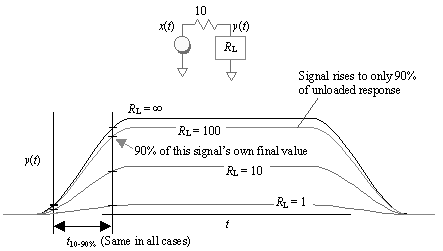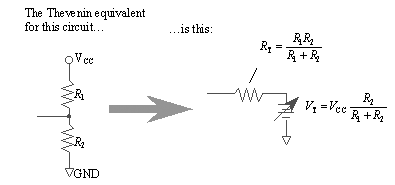# Effect of Split Termination

A resistive load attenuates the output of a digital driver, but does not change its rise (or fall) time. Figure 12.21 illustrates this point with a basic step-response test. A digital logic gate is represented in the figure by a step voltage source with an output impedance of 10 W . The response y ( t ) is shown for four different values of R L .

Figure 12.21. A resistive load leaves t 10-90% unchanged,With R L set to infinity (an open circuit) the output y ( t ) duplicates x ( t ), rising to a full open-circuit voltage of the driver. Both 10% and 90% crossings are marked on the chart, illustrating the 10% to 90% open-circuit risetime of the source, t 10-90% .

With R L set to 100 ohms the signal does not rise quite so high. With a driver of 10 W and a load R L of 100 W , you should expect to see only a 90% response.  The signal at its peak will therefore only just approach 90% of the open-circuit amplitude. If you define risetime by waiting to see when the signal crosses 90% of the open-circuit voltage, you could be stuck waiting for a very long time indeed. With an 89- W load, for example, you'd never make it ”the risetime would be undefined.

 The exact amplitude should be 100/(100 + 10) times the open-circuit amplitude.

To avoid such difficulties, the risetime of a digital signal is defined as the difference between the times at which the signal crosses 10% and 90% of its own steady-state amplitude . With this definition, the 10% and 90% crossings of the 100- W loaded waveform line up exactly with the 10% and 90% points on the open-circuit waveform.

Load the circuit with 10 W and it cuts the signal in half, yet the 10% and 90% crossings of the half- sized signal line up exactly with the other signals. A 1- W load produces a very small signal indeed, but with the same 10% to 90% risetime as in the other cases.

Resistive loads make the signal smaller but do not change how quickly it moves from one level to another.

What confuses many engineers about this subject is the way resistive loading changes circuit timing. This effect is illustrated in Figure 12.22. Here the signal y ( t ) feeds a digital receiver, which has a fixed switching threshold. As the resistive load R L is changed, the threshold remains fixed, thereby changing the point in time at which the loaded waveform crosses the receiver threshold.

Figure 12.22. A resistive load pulling to ground retards the leading edge but accelerates the falling edge. Resistive loads pulling to V CC have the opposite effect.In this example a load of 10 W to ground retards the switching time associated with the rising edge, but advances the switching time associated with the falling edge. A 10- W resistor to V CC would have the opposite effect. Resistive loads have a definite effect on signal timing, but they do not change the 10% to 90% rise or fall time (or the signal bandwidth).

A split termination can provide an interesting timing adjustment. A split terminator acts in many ways just like a single resistor going to an adjustable battery. The setting of the battery is determined by the ratio R 1 / R 2 . This circuit (Figure 12.23) is called a Thevenin-equivalent circuit for the split termination.

Figure 12.23. Changing the ratio R 1 / R 2 biases a digital signal up or down by a small amount.The Thevenin-equivalent battery voltage may be adjusted up or down by changing the ratio of R 1 / R 2 . The effect of this adjustment is to bias the DC level of digital signal y ( t ) up or down by a small amount. The degree of adjustment obtained depends on the ratio of the termination impedance (parallel combination of R 1 and R 2 ) as compared to the output resistance of the driver.

This DC-bias adjustment is normally made to ensure that the transmitting gate meets its V OH and V OL obligations; however, the same adjustment also is occasionally useful when making very small tweaks to system timing.

POINT TO REMEMBER

• Resistive loading attenuates the output of a digital driver, but does not change its rise (or fall) time.High-Speed Signal Propagation[c] Advanced Black Magic
ISBN: 013084408X
EAN: N/A
Year: 2005
Pages: 163